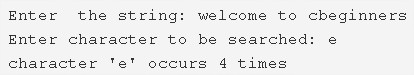# C Program To Count Occurrences Of A Character In String | C Programs

C program to count the occurrences of a character in a given string – In this article, we will discuss the various means to count the occurrences of a character in a given string in C programming.

Suitable examples and sample programs have also been added so that you can understand the whole thing very clearly. The compiler has also been added with which you can execute it yourself.

The means used in this piece are as follows:

• Using Standard Method
• Using Function
• Using Recursion

A string is nothing but an array of characters. The value of a string is determined by the terminating character. Its value is considered to be 0.As you can see in the image uploaded above, firstly, you need to enter the string concerned.

The string here, in this case, is “welcome to cbeginners”.

Later, you have to assign a particular character to be searched in the string.

Character ‘e’ is to be searched in the said example.

Thus, character ‘e’ occurs around 4 times in the whole string.

Hence, the ways to do the same in C programming are as follows:

## Using Standard Method

1. Count=0,Read the entered string as gets(s).

2) Read the entered character c as getchar().

3) Compare the entered character with the elements of the string using for loop with the structure for(i=0;s[i];i++).

If the character match with s[i] then increase the count value by 1.

4) For each occurrence of the character, the count value will be increased. So after all iterations of for loop, we will get total occurrences of the character in the string.

Output:

### Using Function

1. Count=0.

The function check(char *s, char c),

a) Compare the given character with all elements of the string using for loop for(i=0;s[i];i++).

b) If it matches with the element of the string then increase the count value.

c) The function returns the count value to the main() function then main() prints the count value.

Output:

#### Using Recursion

1. The function returns the default count value if there is no element exist in the string.

2) If the string contains elements and s[i] is not null then

The function compares the s[i] with the entered character. If both are equal then it increases the count value.

Increase the i value.

Call the function itself.

The function calls itself recursively until s[i] becomes null then the function returns the count value to the main() function.

3) The main() function prints the count value.

Output:
x

## C Program To Print Number Of Days In A Month | Java Tutoring

C program to input the month number and print the number of days in that ...